Function Repository Resource:

# JacobiShufflePermutations

Generate a set of permutations for Jacobi identities of arbitrary order

Contributed by: Motoharu Ito
 ResourceFunction["JacobiShufflePermutations"][k,l] Find the Jacobi subset of (k,l)-order, where k and l are non-negative integers.

## Details

[X1,,Xn] represents the bracketed product that extends the Lie bracket product called the left-normed bracket, which is defined recursively by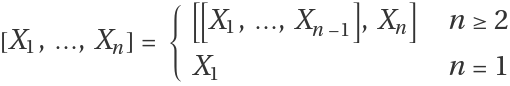For any Lie algebra, a subset J of the symmetric group Sn is said to be a Jacobi subset if it satisfies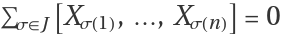. This identity is also called the n th-order Jacobi identity of J.
A Jacobi subset J can be labeled Jk,l for k+ln, and Jk,l is called a Jacobi subset of (k,l)-order.
The explicit expression for Jk,l is represented by the following complex expression. Despite its complexity, it can be organized using the shuffle Sh1.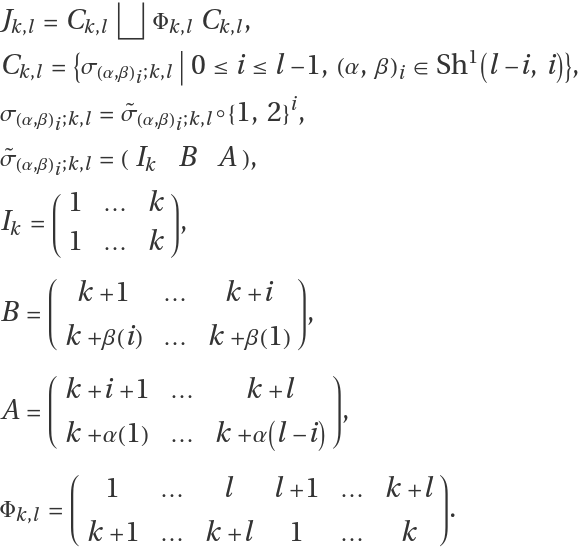For example, since J1,1={{1,2},{2,1}} for n=2=1+1, the second-order Jacobi identity is the anti-commutator. And since J1,2=J2,1={{1,2,3},{2,3,1},{3,1,2}} for n=3=1+2=2+1, the third-order Jacobi version is the standard Jacobi identity.

## Examples

### Basic Examples (3)

Give the anti-commutator ([X1,X2]+[X2,X1]=0) permutations:

 In:=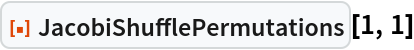Out=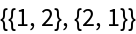Give the Jacobi identity ([X1,X2,X3]+[X2,X3,X1]+[X3,X1,X2]=0) permutations:

 In:=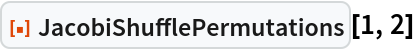Out=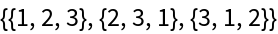Give the higher Jacobi identity ([X1,X2,X3,X4]+[X1,X4,X3,X2]+[X2,X3,X4,X1]+[X3,X1,X2,X4]+[X4,X1,X2,X3]=0) permutations:

 In:=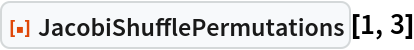Out=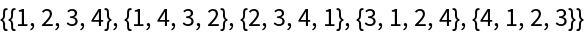### Scope (3)

Prove the Jacobi identity for a matrix:

 In:=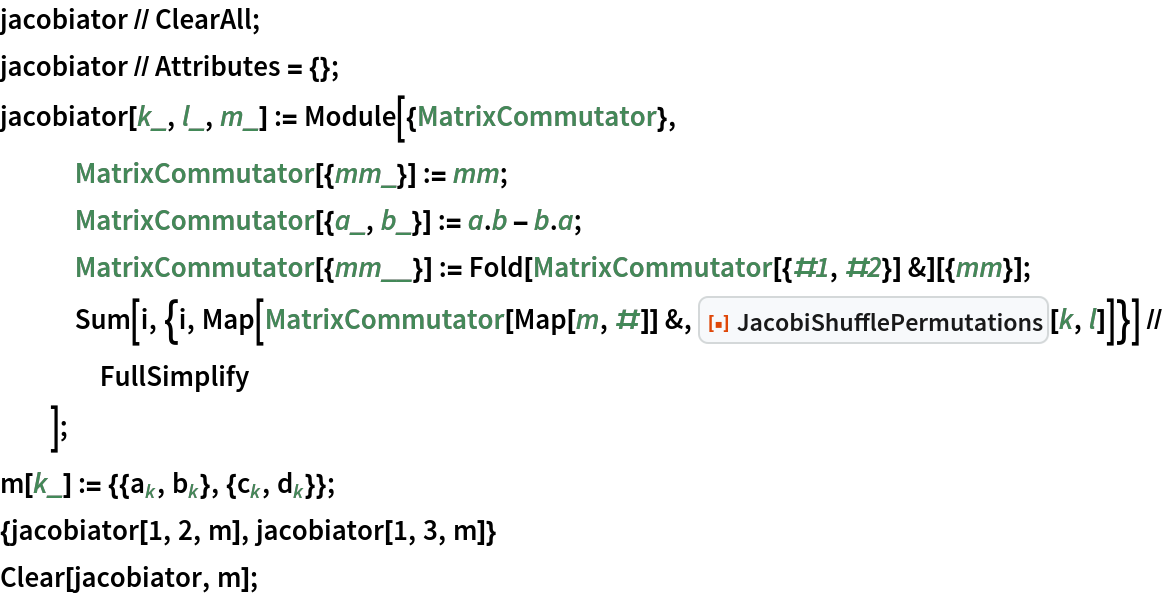Out=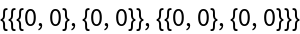Visualize the (1,3)-order Jacobi identity with ResourceFunction[“PermutationCyclesGraph”]:

 In:=Out=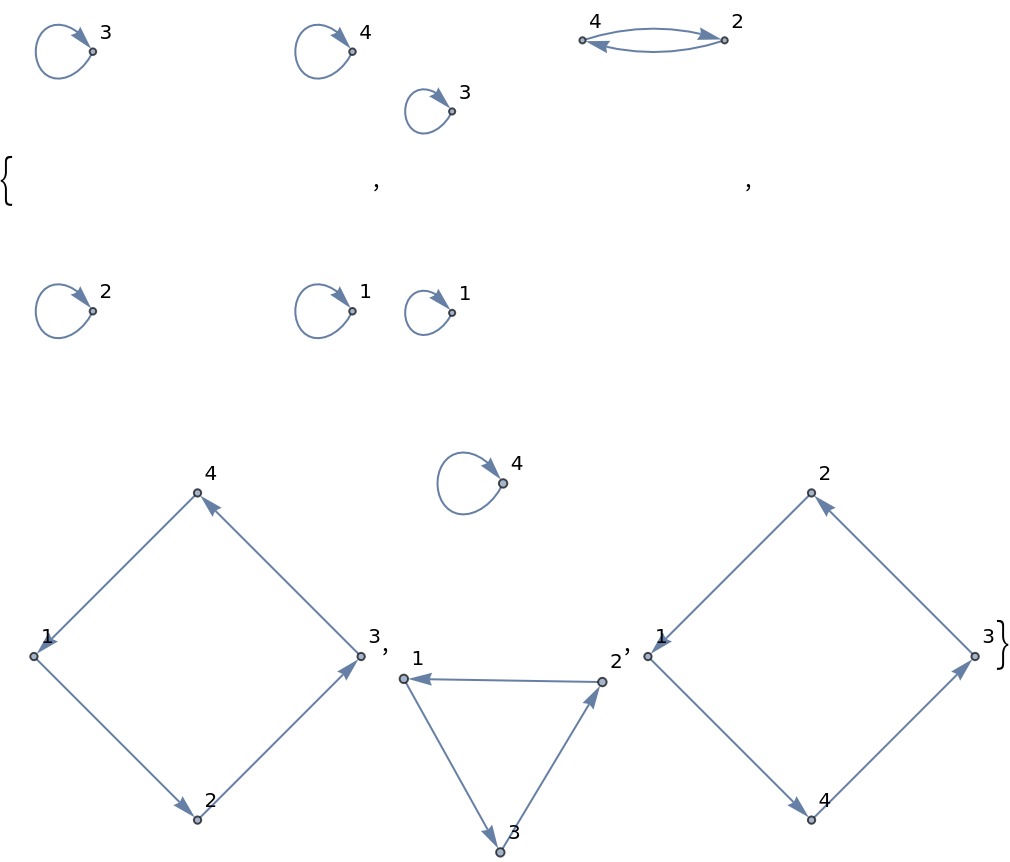Visualize the (3,3)-order Jacobi identity with ResourceFunction[“PermutationCyclesGraph”]:

 In:=Out=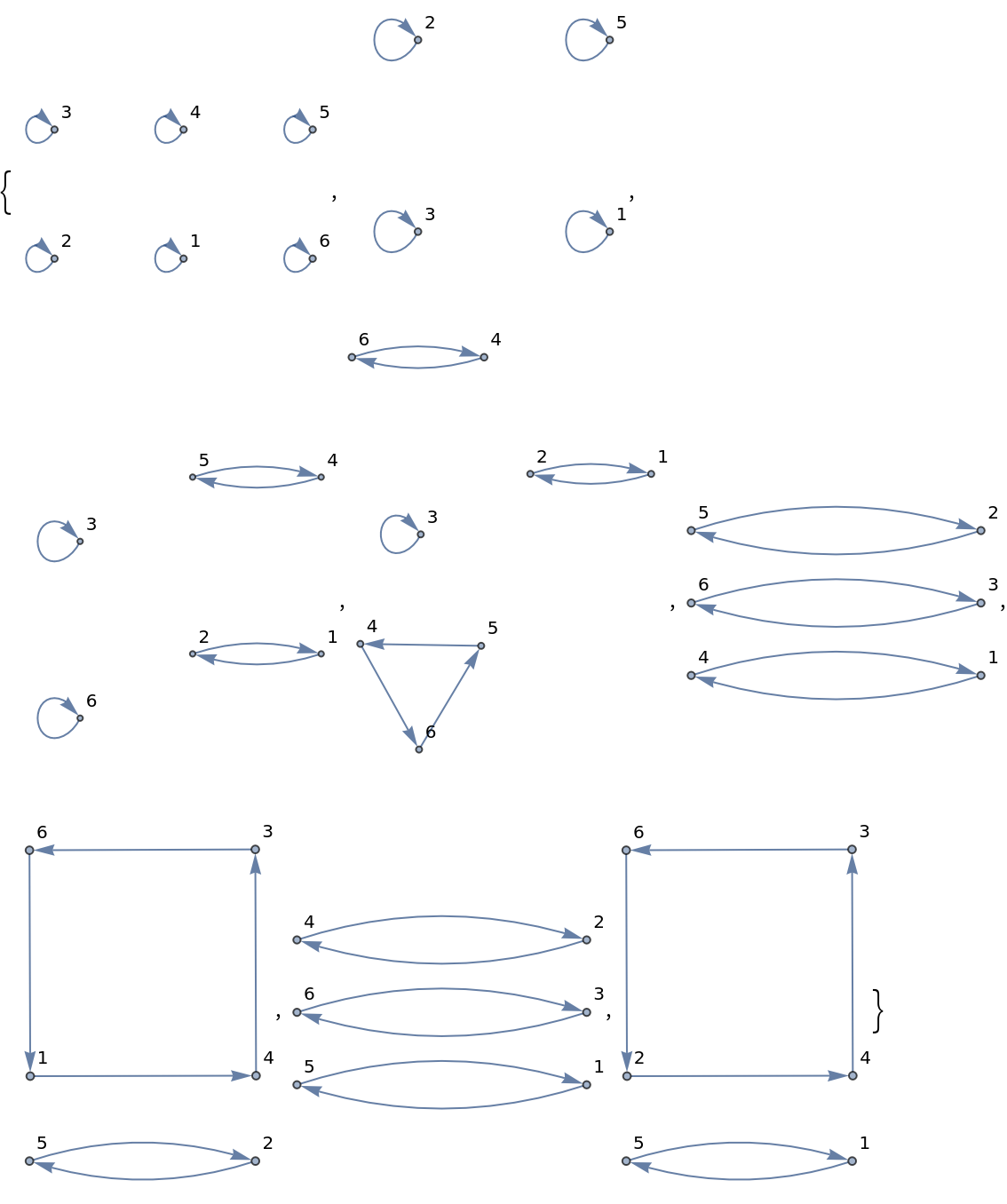Motoharu Ito

## Version History

• 1.0.0 – 14 November 2022